AP State Board Syllabus AP SSC 10th Class Maths Textbook Solutions Chapter 11 Trigonometry Ex 11.4 Textbook Questions and Answers.

## AP State Syllabus SSC 10th Class Maths Solutions 11th Lesson Trigonometry Exercise 11.4

### 10th Class Maths 11th Lesson Trigonometry Ex 11.4 Textbook Questions and Answers

Question 1.
Evaluate the following:
i) (1 + tan θ + sec θ) (1 + cot θ – cosec θ)
Given (1 + tan θ + sec θ) (1 + cot θ – cosec θ)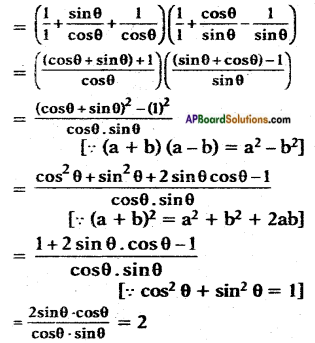ii) (sin θ + cos θ)2 + (sin θ – cos θ)2
Given (sin θ + cos θ)2 + (sin θ – cos θ)2
= (sin2 θ + cos2 θ + 2 sin θ cos θ) + (sin2 θ + cos2 θ – 2 sin θ cos θ) [∵ (a + b)2 = a2 + b2 + 2ab
(a – b)2 = a2 + b2 – 2ab]
= 1 + 2 sin θ cos θ + 1 – 2 sin θ cos θ [∵ sin2 θ + cos2 θ = 1]
= 1 + 1
= 2

iii) (sec2 θ – 1) (cosec2 θ – 1)
Given (sec2 θ – 1) (cosec2 θ – 1)
= tan2 θ × cot2 θ [∵ sec2 θ – tan2 θ = 1; cosec2 θ – cot2 θ = 1]
= tan2 θ × $$\frac{1}{\tan ^{2} \theta}$$ = 1Question 2.
Show that (cosec θ – cot θ)2 = $$\frac{1-\cos \theta}{1+\cos \theta}$$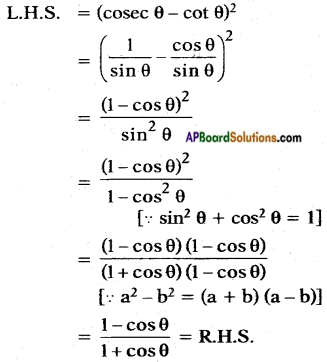Question 3.
Show that $$\sqrt{\frac{1+\sin A}{1-\sin A}}$$ = sec A + tan A
Given that L.H.S. = $$\sqrt{\frac{1+\sin A}{1-\sin A}}$$
Rationalise the denominator, rational factor of 1 – sin A is 1 + sin A.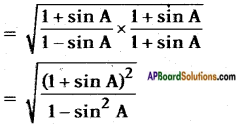[∵ (a + b)(a + b) = (a + b)2]; (a – b)(a + b) = a2 — b2]
= $$\sqrt{\frac{(1+\sin A)^{2}}{\cos ^{2} A}}$$
= $$\frac{1+\sin A}{\cos A}$$
= $$\frac{1}{\cos A}+\frac{\sin A}{\cos A}$$
= sec A + tan A = R.H.S.Question 4.
Show that $$\frac{1-\tan ^{2} A}{\cot ^{2} A-1}$$ = tan2 A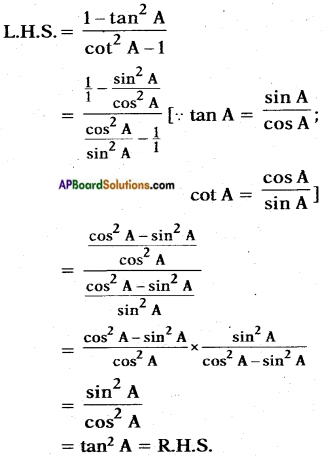Question 5.
Show that $$\frac{1}{\cos \theta}$$ – cos θ = tan θ – sin θ.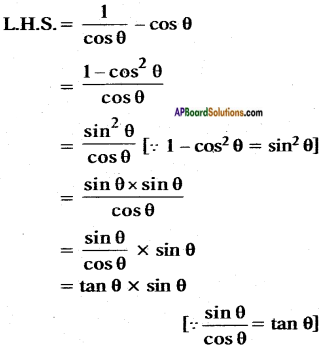Question 6.
Simplify sec A (1 – sin A) (sec A + tan A)
L.H.S. = sec A (1 – sin A) (sec A + tan A)
= (sec A – sec A . sin A) (sec A + tan A)
= (sec A – $$\frac{1}{\cos A}$$ . sin A) (sec A + tan A)
= (sec A – tan A) (sec A + tan A)
= sec2 A – tan2 A [∵ sec2 A – tan2 A = 1]
= 1

Question 7.
Prove that (sin A + cosec A)2 + (cos A + sec A)2 = 7 + tan2 A + cot2 A
L.H.S. = (sin A + cosec A)2 + (cos A + sec A)2
= (sin2 A + cosec2 A + 2 sin A . cosec A) + (cos2 A – sec2 A + 2 cos A . sec A) [∵ (a + b)2 = a2 + b2 + 2ab]
= (sin2 A + cos2 A) + cosec2 A + 2 sin A . $$\frac{1}{\sin A}$$ + sec2 A + 2 cos A . $$\frac{1}{\cos A}$$
[∵ $$\frac{1}{\sin A}$$ = cosec A; $$\frac{1}{\cos A}$$ = sec A]
= 1 +(1 + cot2 A) + 2 + (1 + tan2 A) + 2
[∵ sin2 A + cos2 A = 1; cosec2 A = 1 + cot2 A; sec2 A = 1 + tan2 A]
= 7 + tan2 A + cot2 A
= R.H.S.

Question 8.
Simplify (1 – cos θ) (1 + cos θ) (1 + cot2 θ)
Given that
(1 – cos θ) (1 + cos θ) (1 + cot2 θ)
= (1 – cos2 θ) (1 + cot2 θ)
[∵ (a – b) (a + b) = a2 – b2]
= sin2 θ. cosec2 θ [∵ 1 – cos2 θ = sin2 θ; 1 + cot2 θ = cosec2 θ]
= sin2 θ . $$\frac{1}{\sin ^{2} \theta}$$ [∵ cosec θ = $$\frac{1}{\sin \theta}$$]
= 1Question 9.
If sec θ + tan θ = p, then what is the value of sec θ – tan θ?
Given that sec θ + tan θ = p ,
We know that sec2 θ – tan2 θ = 1
sec2 θ – tan2 θ = (sec θ + tan θ) (sec θ – tan θ)
= p (sec θ – tan θ)
= 1 (from given)
⇒ sec θ – tan θ = $$\frac{1}{p}$$

Question 10.
If cosec θ + cot θ = k, then prove that cos θ = $$\frac{k^{2}-1}{k^{2}+1}$$
Method-I:
Given that cosec θ + cot θ = k
R.H.S. = $$\frac{k^{2}-1}{k^{2}+1}$$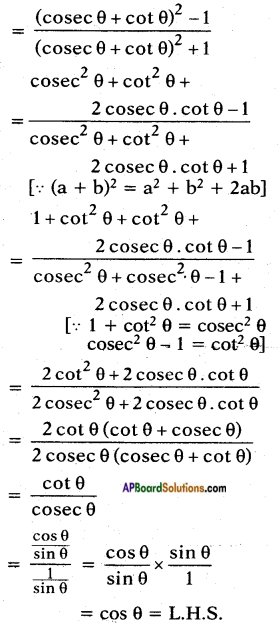Method – II:
Given that cosec θ + cot θ = k ……..(1)
We know that cosec2 θ – cot2 θ = 1
⇒ (cosec θ + cot θ) (cosec θ – cot θ) = 1 [∵ a2 – b2 = (a -b)(a + b)]
⇒ k (cosec θ – cot θ) = 1
⇒ (cosec θ – cot θ) = $$\frac{1}{k}$$
By solving (1) and (2)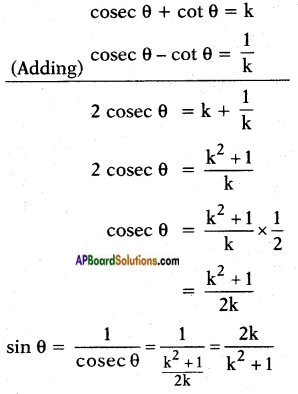According to identity cos2 θ + sin2 θ = 1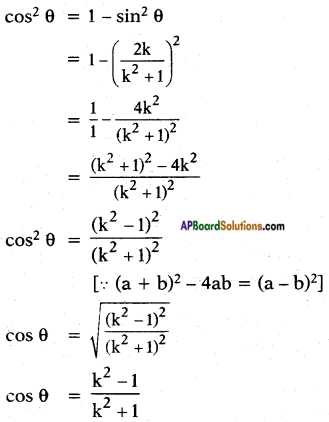Hence proved.